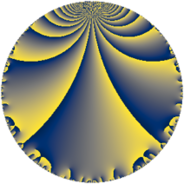# Properties

 Label 27.4.cLevel $27$ Weight $4$ Character orbit 27.c Rep. character $\chi_{27}(10,\cdot)$ Character field $\Q(\zeta_{3})$ Dimension $4$ Newform subspaces $1$ Sturm bound $12$ Trace bound $0$

# Related objects

## Defining parameters

 Level: $$N$$ $$=$$ $$27 = 3^{3}$$ Weight: $$k$$ $$=$$ $$4$$ Character orbit: $$[\chi]$$ $$=$$ 27.c (of order $$3$$ and degree $$2$$) Character conductor: $$\operatorname{cond}(\chi)$$ $$=$$ $$9$$ Character field: $$\Q(\zeta_{3})$$ Newform subspaces: $$1$$ Sturm bound: $$12$$ Trace bound: $$0$$

## Dimensions

The following table gives the dimensions of various subspaces of $$M_{4}(27, [\chi])$$.

Total New Old
Modular forms 24 8 16
Cusp forms 12 4 8
Eisenstein series 12 4 8

## Trace form

 $$4q + 3q^{2} - 5q^{4} + 15q^{5} - 7q^{7} - 66q^{8} + O(q^{10})$$ $$4q + 3q^{2} - 5q^{4} + 15q^{5} - 7q^{7} - 66q^{8} + 12q^{10} + 66q^{11} + 11q^{13} + 60q^{14} + 7q^{16} - 198q^{17} - 154q^{19} - 12q^{20} + 33q^{22} + 33q^{23} + 121q^{25} + 528q^{26} + 332q^{28} - 51q^{29} - 43q^{31} - 423q^{32} - 297q^{34} - 6q^{35} - 100q^{37} - 561q^{38} - 264q^{40} + 132q^{41} - 88q^{43} + 462q^{44} - 528q^{46} + 399q^{47} + 513q^{49} - 429q^{50} + 770q^{52} - 108q^{53} + 1254q^{55} + 66q^{56} + 60q^{58} + 798q^{59} - 439q^{61} - 228q^{62} - 1454q^{64} + 165q^{65} - 988q^{67} + 693q^{68} - 318q^{70} - 2736q^{71} - 910q^{73} + 816q^{74} + 1529q^{76} - 165q^{77} + 803q^{79} - 192q^{80} + 3630q^{82} + 813q^{83} - 594q^{85} + 33q^{86} - 1221q^{88} + 792q^{89} - 1562q^{91} - 858q^{92} - 2100q^{94} - 132q^{95} - 736q^{97} + 846q^{98} + O(q^{100})$$

## Decomposition of $$S_{4}^{\mathrm{new}}(27, [\chi])$$ into newform subspaces

Label Dim. $$A$$ Field CM Traces $q$-expansion
$$a_2$$ $$a_3$$ $$a_5$$ $$a_7$$
27.4.c.a $$4$$ $$1.593$$ $$\Q(\sqrt{-3}, \sqrt{-11})$$ None $$3$$ $$0$$ $$15$$ $$-7$$ $$q+(\beta _{1}+\beta _{3})q^{2}+(-4+\beta _{1}-3\beta _{2}+3\beta _{3})q^{4}+\cdots$$

## Decomposition of $$S_{4}^{\mathrm{old}}(27, [\chi])$$ into lower level spaces

$$S_{4}^{\mathrm{old}}(27, [\chi]) \cong$$ $$S_{4}^{\mathrm{new}}(9, [\chi])$$$$^{\oplus 2}$$